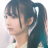## Where communities thrive

• Join over 1.5M+ people
• Join over 100K+ communities
• Free without limits
##### People
•••••••••••••••••##### ActivityHuayi Wei
@weihuayi
(x^2 + y^2=1)
( x^2 )
$x ^2$Huayi Wei
@weihuayi
$x^2 = 1$
$\sum_{i=0}i$Dini0917
@Dini0917
注册成功了Huayi Wei
@weihuayi
$\| \nabla u - \nabla u_h\|_0$
$\| \nabla u - G(\nabla u_h) + G(\nabla u_h) - \nabla u_h\|$Xpangz
@Xpangz
老师，我有个问题，题目的式子是$-u''(x)+u(x)=f(x)$,我对$u''(x)$这里进行差分逼近，带入到式子中，$u(x_i)$的系数不就是$1-\dfrac{1}{h^2}$吗，带入计算是第二幅图，而用之前的系数$-\dfrac{1}{h^2}$，图像就是第一幅图，很逼近，请问这是什么问题？Huayi Wei
@weihuayi
@Xpangz 这应该是右端没计算对的问题， 你模型的右端 $f$ 等于 $-u''$， 而不是 $-u'' + u$ .
另外， 输入公式 $$-u''(x) + u(x) = f(x)$$
数学公式应该放到双美元符号的中间Xpangz
@Xpangz
嗯嗯，我知道我自己错在哪了，已改正，谢谢魏老师！！zhangtong0616
@zhangtong0616
注册成功，大家好，开始学习FatHappier-Pang
@FatHappier-Pang
老师，微分方程数值解目录有个地方打错了，一维激波管问题打成一维管激波了Huayi Wei
@weihuayi
好的，我修改一下W. Wang
@Geniuswangwei
请问老师,当我利用Fealpy计算出双旋度问题(时谐Maxwell)数值解$u_h$, 我应该如何获取数值解$u_h$的图像? 特别是双旋度问题的真解是一个$2 \times 1$的向量, 那么我的uh的图像如何体现?
我利用的是fealpy中第一类棱元空间Huayi Wei
@weihuayi
你可以用 matplotlib 中的 quiver 函数来画图
@GeniuswangweiW. Wang
@Geniuswangwei
好的, 我试试, 谢谢魏老师(^▽^)魏老师, 您好, 还是之前的问题, 利用matplotlib.quiver能画出真解的矢量场图, 那数值解的呢? 数值解是一个一维向量, 而真解是一个$2 \times 1$的向量
@weihuayi想请教一下, 这种图在matplotlib怎么画, 原图是ifem中showsolution画的, 此外fealpy中是否有类似功能?Huayi Wei
@weihuayi